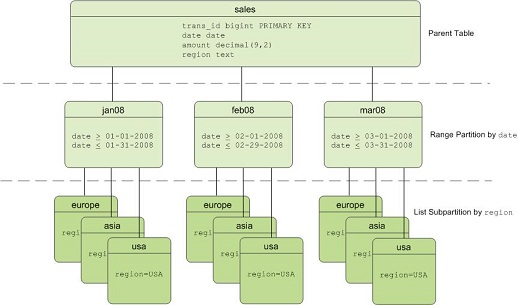A large table can be divided into smaller storage units called partitions. If you specify query criteria, only the data in partitions that meet the criteria is scanned. This improves query performance.

## Table partitioning types supported

• Range partitioning: Data is divided based on a numerical range, such as date.
• List partitioning: Data is divided based on a list of values, such as city attributes.
• Multi-level partitioning: Data is divided based on both a numerical range and a list of values.The preceding figure shows an example of a multi-level partitioned table. Data in level-1 partitions is divided by month, and data in level-2 partitions is divided based on the values of regions.

## Range partitioned tables

You can have AnalyticDB for PostgreSQL automatically generate partitions by assigning a START value, an END value, and an EVERY clause that defines the partition increment value. By default, START values are always inclusive and END values are always exclusive. Example:

``````CREATE TABLE sales (id int, date date, amt decimal(10,2))
DISTRIBUTED BY (id)
PARTITION BY RANGE (date)
( START (date '2016-01-01') INCLUSIVE
END (date '2017-01-01') EXCLUSIVE
EVERY (INTERVAL '1 day') );``````

You can create a range partitioned table that uses a column of a numeric data type as the partition key. Example:

``````CREATE TABLE rank (id int, rank int, year int, gender char(1), count int)
DISTRIBUTED BY (id)
PARTITION BY RANGE (year)
( START (2006) END (2016) EVERY (1),
DEFAULT PARTITION extra ); ``````

## List partitioned tables

A list partitioned table can use any data type column that allows equality comparisons as a partition key. For list partitions, you must declare a partition specification for every partition (list value) that you want to create. Example:

``````CREATE TABLE rank (id int, rank int, year int, gender
char(1), count int )
DISTRIBUTED BY (id)
PARTITION BY LIST (gender)
( PARTITION girls VALUES ('F'),
PARTITION boys VALUES ('M'),
DEFAULT PARTITION other );``````

## Multi-level partitioned tables

You can create a table with multi-level partitions. The following example shows a three-level partition design. Data in level-1 partitions is divided by month, and data in level-2 partitions is divided based on the values of regions.
``````CREATE TABLE sales
(id int, year int, month int, day int, region text)
DISTRIBUTED BY (id)
PARTITION BY RANGE (month)
SUBPARTITION BY LIST (region)
SUBPARTITION TEMPLATE (
SUBPARTITION usa VALUES ('usa'),
SUBPARTITION europe VALUES ('europe'),
SUBPARTITION asia VALUES ('asia'),
DEFAULT SUBPARTITION other_regions)
(START (1) END (13) EVERY (1),
DEFAULT PARTITION other_months );``````

## Granularity of table partitioning

For a table partitioned by time, the granularity can be a day, week, or month. A finer granularity results in a smaller amount of data in each partition but a larger number of partitions. The number of partitions is not measured by an absolute standard. In general, about 200 partitions are plentiful in number. A large number of partitions has a significant impact on database performance. For example, it slows operations of the query optimizer and many maintenance operations such as VACUUM.

## Optimization of queries on partitioned tables

AnalyticDB for PostgreSQL supports partition truncating for partitioned tables. Only required partitions are scanned based on query criteria, which improves query performance. Example:
``````explain
select * from sales
where year = 2008
and  month = 1
and  day = 3
and region = 'usa';``````

The query criteria fall on the level-3 subpartition 'usa' of the level-2 subpartition 1 of level-1 partition 2008. Therefore, only the data in the level-3 subpartition 'usa' is scanned during the query. As shown in the following query plan, only one of the 468 level-3 subpartitions is read.

``````Gather Motion 4:1  (slice1; segments: 4)  (cost=0.00..431.00 rows=1 width=24)
->  Sequence  (cost=0.00..431.00 rows=1 width=24)
->  Partition Selector for sales (dynamic scan id: 1)  (cost=10.00..100.00 rows=25 width=4)
Filter: year = 2008 AND month = 1 AND region = 'usa'::text
Partitions selected:  1 (out of 468)
->  Dynamic Table Scan on sales (dynamic scan id: 1)  (cost=0.00..431.00 rows=1 width=24)
Filter: year = 2008 AND month = 1 AND day = 3 AND region = 'usa'::text``````

## Partition definition query

You can use the following SQL statement to query the definitions of all partitions in a table:
``````SELECT
partitionboundary,
partitiontablename,
partitionname,
partitionlevel,
partitionrank
FROM pg_partitions
WHERE tablename='sales';``````

## Maintenance of partitioned tables

Partitioned tables support diverse partition-related operations such as add, drop, rename, clear, swap, and split partitions. For more information, visit CREATE TABLE.